All submissions of the EM system will be redirected to Online Manuscript Submission System. Authors are requested to submit articles directly to Online Manuscript Submission System of respective journal.

# Original Article

, Volume: 6( 1)

## On New Foundations of Theory of Atom

*Correspondence:
Temur ZK
Department of Physical Problems, Yozuvchilar, Tashkent, Uzbekistan, Tel: +86-0532-84022681; E-mail: tzk_uz@yahoo.com.

Received Date: February 02, 2018 Accepted Date: March 21, 2018 Published Date: March 28, 2018

Citation: Temur ZK. On New Foundations of Theory of Atom. J Phys Astron. 2018; 6(1):144

### Abstract

The correct approach to the formulation of a new basis of quantum theory and new foundations of theory of atom is proposed. The unity of formal logic and rational dialectics is the correct methodological basis of the approach. The main result is the following principles: (1) the principle of motion of quantum particle; the principle of stability of quantum particle; (3) principle of energy of quantum particle; (4) the principle of speed of translational motion of quantum particle; (5) the principle of rectilinearity of the external (translational) motion of quantum particle; (6) the principle of mass of quantum particle; (7) the principle of acceleration and of deceleration of quantum particle; (8) the principle of translational motion of electron in atom: the translational motion of electron in atom represents the periodical “scattering” of the electron by nucleus; (9) the principle of translational motion of electrons in many-electron atom: the translational motion of electrons in many-electron atom does not lead to formation of the electron shells. The proposed foundations furnish the clue to correct description of reality.

### Keywords

Foundations of theoretical physics; Foundations of quantum mechanics; Mathematical physics; Atomic and molecular physics; General laser theory; Quantum electronics; Formal logic; Philosophy of science

PACS: 01.55.+b, 01.65.+g, 03.65.-w, 03.65.Ta, 31.10.+z, 31.15.-p, 32.10.-f, 42.55.-f, 42.55.Ah e

### Introduction

As is well known, quantum (wave) mechanics is a fundamental theory in physics which describes the nature of the smallest scales of energy levels of atoms and subatomic particles. Important applications of quantum mechanics include quantum chemistry, superconducting magnets, light-emitting diodes, and the laser, the transistor and semiconductors such as the microprocessor, medical and research imaging such as magnetic resonance imaging and electron microscopy. Explanations for many biological and physical phenomena are rooted in the nature of the chemical bond, most notably the macro-molecule.

As is known, quantum (wave) mechanics was created in 1990-1927 by outstanding physicists: M. Planck, A. Einstein, A. Compton, L. de Broglie, C. Davisson, L. Germer, E. Schrödinger, P. Dirac, M. Born, N. Bohr, W. Heisenberg, W. Pauli, etc. But the scientific works of the classics of physics and modern well-known physicists [1-15] do not rest on the correct methodological basis: the unity of formal logic and rational dialectics. This fact allows independent researchers to question the achievements of quantum (wave) mechanics.

The great successes achieved by quantum (wave) mechanics are undeniable [1-15], are not they? But these successes do not represent a proof of the validity (truth) of quantum (wave) mechanics. Great physicists (for example, N. Bohr) could not refute Einstein’s criticisms (and opinion): “The more successes are achieved by quantum theory, the more stupid it looks. How people far from physics would laughed if they knew about this state of the art”. “The great successes achieved by quantum theory… cannot hide from us the fact that the logical basis of this theory is absent”. In finding a common basis for all physics, one must, in my opinion, be careful to base oneself dogmatically upon scheme of the modern theory”. “Perfection of the mathematical formalism of the theory and its considerable success hidden from our view the weight of those sacrifices which have been made for this success”. Einstein could not prove his assertions because he did not find the correct methodological basis for a correct scientific analysis of the foundations of quantum (wave) mechanics. What is more, all outstanding physicists could not prove the validity (truth) or falsity of the foundations of quantum (wave) mechanics [1-15]. In the works [16-38], it is proved within the framework of the correct methodological basis (i.e., the unity of formal logic and rational dialectics) that the foundations of quantum (wave) mechanics are incorrect because they contain the following main errors: the standard interpretation of the experimental data on the diffraction of the quantum particles; the concept of wavecorpuscle dualism; the probabilistic interpretation of square of module of the psi-function. The formal-logical basis for a new quantum theory was proposed in works [16-32,37]. The purpose of this work is to propose new principles of construction of the theory of atom within the framework of the correct methodological basis.

### A New Basis of Quantum Theory

The new basis of quantum theory was proposed for the first time in works [16-32,37]. It represents a new viewpoint that has arisen from the critical analysis of statistical physics, the special theory of relativity, and standard quantum mechanics. The basis is formed by the following heuristic principles: The principle of motion of quantum particle is as follows: the motion of quantum particle is the form of existence of quantum particle; the motion represents unity of internal and external (i.e. translational) motions. (Why?) The principle of stability of a quantum particle is as follows: a free quantum particle is identical with itself and has an invariable quantitative determinacy for unlimited time (in the course of unrestrictedly long time). In other words, the quantitative and qualitative determinacy of a free (stable) quantum particle is conserved in the course of unrestrictedly long time. (Why?) The principle of energy of quantum particle is as follows: the energy,(Where is the energetic quantum number which characterizes the quantitative determinacy of the particle) is inalienable property of a quantum particle. The energy levels of the quantum particle arise and disappear only as a result of absorption and of emission of other quantum particles (photons, ???????????? ??????), respectively. (Consequently, the problem of quantization of energy is not the Schrödinger problem of eigenvalues The dimension of the energy of a quantum particle is . From the point of view of formal logic, this is the correct dimension of the kinetic (external) energy of a particle. (Why?) 4) De Broglie’s empirical formula for photon (according to which relation between corpuscular and wave aspects of motion of a quantum particle is established by the mathematical equation where is the energy of the particle, is the frequency of the wave, is the Planck constant) can be corrected and generalized in the following way. The principle of equivalence of energy and frequency of quantum particle is as follows: energy is connected with frequency by the following relationship: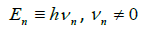Where and are the Planck ‘constant’ (i.e. universal quantum of action) and the frequency of the periodic process of mutual transformation of the internal and external motions of particle, respectively. From the point of view of formal logic, this relationship is correct. The concepts of energy and of frequency are identical ones. Multiplication of the quantities and is permitted by formal-logic law of identity if is an oscillating quantity [16-32,37]. The physical quantities represent the quantities bounded below and above. (Why?) The principle of speed of the translational motion of quantum particle is as follows: the speed is defined by the formula,Whereis the size (the diameter) of the particle (Figure 1).

Figure 1: The scheme of the translational motion as a transformation of microparticle for one oscillation period where is size (diameter) of the microparticle for one oscillation period.

The λn equals the distance traveled by the particle for the oscillation period,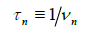This translational motion represents the oscillation contraction (decrease) and extension (increase) of the size (diameter) of the particle. Therefore, the translational motion of the quantum particle relative to a reference system is an absolute one. The absolute motion is invariant under choice of a reference system. This statement means that the velocity addition theorem for quantum particle is not valid. The physical quantities represent the quantities bounded below and above (Why?).

The principle of rectilinearity of the external motion of a quantum particle is as follows: a free (stable) quantum particle moves along a straight line.

The principle of mass and momentum of quantum particle is as follows: the motion mass and the momentum are defined by the formula,This formula is a consequence of the dimension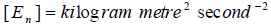(Note: A quantum particle has no rest mass because a quantum particle cannot rest). The concept of mass mn and the concept of energy are not identical ones. Therefore, the formula,does not express the principle of equivalency of mass and energy. The formula is a consequence of the dimensionThe physical quantities represent the quantities bounded below and above.

(Why?)

8) In the case of a photon,Therefore,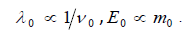Consequently, λ0 is a bounded quantity. (Why?)

9) Formulation of the principle of equivalency of mass and energy of quantum particle would be feasible one if the energy En was connected with the mass Mn by the formulawhere the concepts of the energy En and the mass Mn were identical ones, k was a universal constant,But this formula is incorrect one because the coefficient k represents macroscopic quantity. The physical quantities represent the quantities bounded below and above (Why?).

10) The principle of acceleration and of deceleration of quantum particle is as follows: acceleration and deceleration of particle are results of absorption and emission of other quantum particles (elementary particle), respectively. The acceleration, of the quantum particle under the transitionwhich is effect of absorption of other quantum particle (photon) is defined by the formula,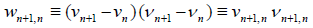The physical quantities represent the quantities bounded below and above. (Why?)

The Principles of New Theory of Atom

The principles of new theory of atom can be constructed within the framework of the formulated basis in the following way. Atom as a microparticle is a physical system of connected microparticles (elements): nucleus and electrons. The connection between the elements (i.e., the structure of the system) is determined by the following electrical forces: (a) the force of attraction between the opposite charges of the nucleus and the electron; (b) the repulsive force between electrons. The connection between nucleus and electrons does not mean the loss of individuality (i.e., qualitative certainty) of the electron and the nucleus.

The translational motions of atom, nucleus and electrons represent the absolute movement. This means that the electron is not on the nucleus and is not absorbed by the nucleus: the electron in the atom moves near the nucleus because the absolutely moving electron is “scattered” (“reflected”) by the nucleus. In other words, the translational motion of the electron is periodic “scattering” of the electron by the nucleus because the absolute motion of electron is an indestructible motion. (The following analogy can be useful for understanding: the ball falls on a sticky surface and is reflected from this surface). The “trajectory” of the motion of the electron in the atom is a closed periodic line Figure 2.

Figure 2: The scheme of the translational motion of electrons in atom where is the nucleus of the atom, the dashed lines depict (represent) the ?trajectories? of unexcited and excited electrons.

The energyThe energyof the atom, the energyof the nucleus of the atom, the energy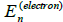of the electron of the atom are defined by the following common relationship: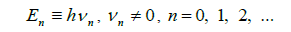The energy state of microparticle (atom, nucleus, electron) under n = 0 is called the unexcited state of the microparticle. The energy state of microparticle under n > 0 is called the excited state of the microparticle.

The excitation of the energy state of electron of atom leads to a change in the binding energy of the electron (i.e., to a change in the structure of the atom) and, consequently, to the excitation of the energy state of the atom. But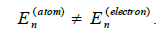The variables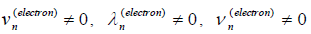are the quantities bounded below and above under the permissible values of n . The quantities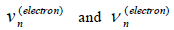are monotonically increasing quantities under increase of n , and the quantityis monotonically decreasing quantity under increase of n in the relationship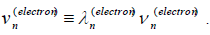This is conformed to the fact that, in the particular case of photon, the following relationship is valid:### Methods

The “trajectory” of the motion of excited electron in atom is farther from the nucleus than the “trajectory” of the motion of unexcited electron in atom becauseThe motion of excited electron in atom can represent the process of inelastic scattering which is accompanied by the emission of photon. The elementary act of emission of photon is described by the following physical relationship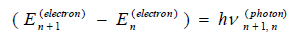where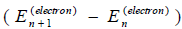is an indecomposable expression. This correct relationship determines the qualitative and quantitative determinacy of the emitted photon. This quantitative relationship satisfies the formal-logical law of identity: (qualitative determinacy of photon)=(qualitative determinacy of photon).

The proof of this assertion is as follows. The relationship,means that the quantity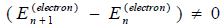does not belong to electron. (In other words, the qualitative determinacy of the quantity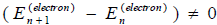does not represent electron). This relationship is a negative formal-logical definition of the concept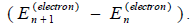A positive (formal-logical, physical, practical) definition of the conceptis the following definition:This definition is correct because it satisfies the formal-logical law of identity.

Remark: But the expression,is incorrect because it does not satisfy the formal-logical law of identity. The excitation of the energy state of the atom (as a system) leads to a change in the energy of bond between the electron and the nucleus. This means a change in the structure of the atom. The atom makes transition from the excited state to the unexcited state by a structural (energy) transition with the emission of photon. The elementary act of structural radiation of the photon by the atom is described by the following relationship:The translational motion of electrons in many-electron atom does not lead to the formation of electron shells (such as K-shell, L-shell, etc.). The electronic “surface” of the atom “looks” like a surface of boiling water.

#### Results and Discussion

Theories can be divided into two classes: theories of the essence; and the theory of the phenomenon (i.e., theory of the manifestation of the essence). Theories of the essence (i.e., the essence of the world) can be created only in a verbal and written (formally-logical) forms and cannot be formulated in mathematical (quantitative) form. Theories of the phenomenon (of the manifestation of the essence of the world) represent phenomenological theories (i.e., theories of the qualitative and quantitative determinacy of phenomena). Physical theories represent phenomenological theories and are divided into two subclasses: correct theories (which are formulated within the framework of the correct methodological basis) and incorrect theories (which contain methodological errors). Elimination of methodological errors from the theory leads to the abolition of the theory.

Standard quantum (wave) mechanics represents an erroneous phenomenological theory because it contains methodological errors. As has already been shown for the first time in works [16-32,37], the relationships.are, in the formal-logical point of view, the only correct relationships in standard quantum mechanics for 100 years of its existence. The principles of the theory of atom proposed above represent the correct concretization of the relationship.(if this relationship is correct in the physical point of view). Obviously, this relationship is a necessary but insufficient condition for constructing the complete phenomenological theory of the atom. The complete phenomenological theory of the atom could be constructed if new principles were found and formulated mathematically. But this did not happen over 100 years of the existence of quantum (wave) mechanics [1-15]. Probably, the complete phenomenological theory of atom (and of quantum particles in general) cannot be created. In my opinion, there are epistemological limitations (restrictions) determined by nature (essence) and destination of man and mankind [33-36,38]. All standard theories which are consistent with experiment but contradict to formal logic and dialectics contain a fraction of the truth.

Example 1

As is known, in 1941 the Nobel Prize winner in physics (1962) L.D. Landau created the quantum theory of superfluidity of the. The quantum theory of superfluidity explained all known properties of the. But the theory of superfluidity was based on the idea of the false theory of “thermal liquid”. Exactly, the false theory of “thermal liquid” was the idea, the way of LD.

Landau’s thinking. “I will remind you of a theory that once figured in physics. In physics, there once was the theory of “thermal liquid” which never existed in reality. It was assumed that there is a special “thermal liquid” having the following property: if the body is warm, then it means that the body contains more “thermal liquid”; if the body contains less “thermal liquid”, then it means that the body is less warm. “Thermal liquid” is a liquid that was specially invented to explain thermal phenomena. Experiments have shown that “thermal liquid” does not exist in reality, and heat is motion of particles of a liquid. However, in helium, something has been preserved as the “thermal liquid” in a peculiar sense” (L.D. Landau). In other words, it was assumed that “thermal liquid” is a mythical weightless liquid that exists separately from a given body or liquid; “thermal liquid” is a kind of substance that one can inject into a real liquid (and make a real liquid warmer) or take it away from a real liquid (and make a real liquid less warm). This image suggested L.D. Landau to consider an analogy with “thermal liquid” in explaining the connection of heat with the. The analogy generated the quantum theory of the : the gas of quasi-particles (phonons and rotons) plays the role of the “thermal liquid” in the quantum theory of L.D. Landau.

Thus, the theory of superfluidity would not have been created if the erroneous theory of the “thermal liquid” did not exist.

Example 2

Nobel Laureates in Physics (1964) N.G. Basov, A.M. Prokhorov, Ch. Townes used the Boltzmann distribution to explain the operation of the laser. But, as shown in works [25,27,28,30], the Boltzmann distribution represents a gross statistical and formal-logical error.

Thus, the theory of the laser would not have been created if the Boltzmann distribution did not exist.

All standard theories contain only a fraction of the truth. The fraction of truth, which is contained in standard theories, is conformed to experiment. As great scientists say in this cases, “the theories work”. A scientific lie, which is contained in standard theories, leads to an expansion of the consciousness of all the scientists. If the fraction of truth, which is contained in standard theory, is embodied in material devices (machines, spacecrafts, the atomic and nuclear bombs, etc.), then the fraction of theoretical truth turns into independent, separate, practical, non-theoretical truth.

The science of phenomenon (for example, physics) does not lead to the cognition of the essence because the science of phenomenon represents the inductive (infinite) process of “negation of negation”. Negation of the old scientific lie will create a new scientific lie. The new scientific lie will contain a fraction of a new scientific truth if the correct paradigm of science is not guessed. The correct paradigm of science can be guessed if lie shocks scientists.

#### Conclusion

Thus, the foundations of the generally accepted quantum mechanics contain the following logical errors: the standard interpretation of the experimental data on diffraction of quantum particles; the concept of wave-corpuscle dualism; the probabilistic interpretation of the psi-function. The true meaning of the psi-function is a pseudo-informational meaning. Therefore, quantum mechanics is a nonphysical, nonobjective, and pseudo-informational theory. The incomplete description of objective reality by the pseudo-informational theory should be replaced by the physical, objective quantum theory (i.e. by the correct description of objective reality).

The proposed correct approach to the formulation of a new basis of quantum theory and new principles of theory of atom leads to the following results:

The principle of motion of quantum particle is as follows: the motion represents unity of internal and external (i.e., translational) motions.

The principle of stability of quantum particle is as follows: the quantitative and qualitative determinacy of free (stable) quantum particle is conserved in the course of unrestrictedly long time.

The principle of energy of quantum particle is as follows: the energy is inalienable property of a quantum particle. The energy levels of the quantum particle arise and disappear only as a result of absorption and of emission of other quantum particles (photons, elementary particles), respectively. The energy of the quantum particle is proportional to the frequency of the periodic process of mutual transformation of the internal and external motions of particle, respectively.

The principle of speed of the translational motion of the quantum particle is as follows: the speed is defined by the oscillation contraction (decrease) and extension (increase) of the size (diameter) of the particle. Therefore, the translational motion of the quantum particle relative to a reference system is the absolute one. The absolute motion is invariant under choice of a reference system.

The principle of rectilinearity of the external motion of the quantum particle is as follows: a free (stable) quantum particle moves along a straight line.

The principle of mass of quantum particle is as follows: mass of quantum particle is the motion mass; quantum particle has no rest mass because a quantum particle cannot rest. The concept of mass and the concept of energy are not identical ones. There is no relation of equivalence (identity) between mass and energy.

The principle of acceleration and of deceleration of quantum particle is as follows: acceleration and deceleration of particle are results of absorption and emission of other quantum particles (elementary particles), respectively

Atom as a microparticle is a physical system of bound microparticles (elements): nuclei and electrons. The translational motions of the atom, nucleus, and electrons represent the absolute movement. The translational motion of electron in atom is the periodic “scattering” of the electron by nucleus because the absolute motion of electron is an indestructible motion.

The excitation of the energy state of the electron in the atom leads to a change in the binding energy of the electron (i.e., to a change in the structure of the atom) and, consequently, to the excitation of the energy state of the atom. The motion of excited electron in atom can represent the process of inelastic scattering which is accompanied by the emission of photon.

The excitation of the energy state of atom (as a system) leads to a change in the energy of bond between the electron and the nucleus. This means a change in the structure of the atom. The atom makes the transition from the excited state to the unexcited state by the structural (energy) transition with the emission of photon.

The translational motion of electrons in many-electron atom does not lead to the formation of electron shells (such as K-shell, L-shell, etc.). The proposed foundations furnish the clue to correct description of reality.

#### References

Welcome Message

Select your language of interest to view the total content in your interested language

###### Citations : 272

Journal of Physics & Astronomy received 272 citations as per Google Scholar report

#### Indexed In

• Open J Gate
• China National Knowledge Infrastructure (CNKI)
• Cosmos IF
• Directory of Research Journal Indexing (DRJI)
• MIAR
• Secret Search Engine Labs
• Euro Pub
• clarivate-web-of-science-logo-vector
• ICMJE

View More

### 9th International Conference on Physics

Edinburgh, Scotland

### 6th International Conference on Astronomy, Astrophysics and Space Science

Budapest, Hungary

Berlin, Germany

### 9th International Conference on Physics

Edinburgh, Scotland

### 6th International Conference on Astronomy, Astrophysics and Space Science

Budapest, Hungary

### 10th International Conference on Theoretical and Applied Physics